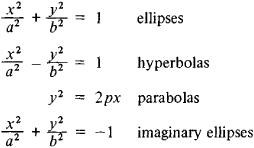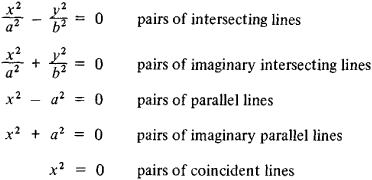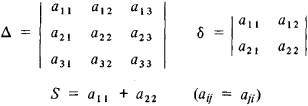The following article is from The Great Soviet Encyclopedia (1979). It might be outdated or ideologically biased.

a plane curve whose rectangular Cartesian coordinates satisfy a second-degree algebraic equation

(*) a11x2 + 2a12xy + a22y2 + 2a13x + 2a23y + a33 = 0

The equation (*) may not define a figure in the real plane. Then, to preserve generality, we say that (*) defines an imaginary quadratic curve. Depending on the values of the coefficients of the general equation (*), it can be transformed by a translation of the origin and a rotation of the coordinate system to one of the nine canonical forms given below, each of which determines a certain class of curves. Namely,

(1) irreducible curves:(2) reducible curves:The nature of quadratic curves can be determined without reducing the general equation to a canonical form. Instead we examine the values of the fundamental invariants of quadratic curves— expressions formed from the terms of equation (*) whose values are not affected by translations and rotations of the coordinate system. The invariants in question areThus, for example, as irreducible curves, ellipses are characterized by the fact that for them Δ ≠ 0; the positive value of the invariant δ differentiates between ellipses and other types of irreducible curves (for hyperbolas, δ < 0; for parabolas, δ = 0). Comparison of the signs of the invariants Δ and S makes it possible to distinguish between real and imaginary ellipses: if Δ and S have different signs, then the ellipse is real; it is imaginary if Δ and S have the same sign.

The three fundamental invariants Δ, δ, and S define quadratic curves (except for parallel lines) to within a motion of the Euclidean plane: if the corresponding invariants Δ, δ, and S of two curves are equal, then these curves can be superposed by a motion. In other words, these curves are equivalent with respect to the group of motions of the plane (they are metrically equivalent).

It is possible to classify quadratic curves relative to other groups of transformations. Thus, any two curves defined by equations of the same canonical form are equivalent with respect to the group of affine transformations that are more general than the group of motions. For example, two similar quadratic curves are affine-equivalent. Classification from the standpoint of projective geometry, in which infinitely remote elements do not play a special role, makes possible the determination of the relations among the different affine classes of quadratic curves. The real irreducible quadratic curves—ellipses, hyperbolas, and parabolas—form one projective class, the class of real oval curves (ovals). A real oval curve is an ellipse, hyperbola, or parabola depending on its position with respect to the line of infinity: an ellipse intersects the ideal line at two imaginary points, a hyperbola intersects it at two different real points, and a parabola is tangent to it: There are projective transformations that send these curves into each other. In all, there are five classes of projectively equivalent quadratic curves. Namely,

(1) nondegenerate curves (x1, x2, x3 are homogeneous coordinates):

x12 + x22x32 = 0 a real oval

x12 + x22 + x32 = 0 an imaginary oval

(2) degenerate curves:

x12x22 = 0 a pair of real lines

x12 + x22 = 0 a pair of imaginary lines

x12 = 0 a pair of coincident real lines

In addition to defining quadratic curves analytically, that is, by means of an equation, we can define them in other ways. For example, the ellipse, hyperbola, and parabola can be obtained as intersections of a cone by a plane, that is, as conic sections.

### REFERENCES

Aleksandrov, P. S. Lektsii po analiticheskoi geometrii.… Moscow, 1968.
Efimov, N. V. Kratkii kurs analiticheskoi geometrii, 5th ed. Moscow, 1960.

A. B. IVANOV